### How to value high growth companies

Fundamentals 26: This post looks at the issues when valuing high-growth companies. I used CBIP to illustrate how to model growth and estimate the intrinsic value.

High-growth companies are those with growth rates several times higher than the long-term GDP growth rate. There are 2 types of growth companies:
• Those coming out of the start-up stage. An example of this is Grab.
• Established companies with sustainable economic moats. An example of this is Coca-cola.

In an earlier post, I presented a financial model to determine the intrinsic value of mature companies. These are companies with growth rates lower than the long-term GDP growth rate.  Refer to "Focusing on the assumptions of perpetual growth model". It is based on a single-stage discounted FCFF model.

You should not use the mature company model to value high-growth companies. Furthermore, you value start-ups differently from established companies with high growth.

Join me as I explore the various approaches for valuing high growth companies and illustrate them with my valuation of CBIP. If you are not familiar with CBIP, I suggest that you first read my article “Is CBIP a money making opportunity? Find out using Shareinvestor.

## Contents

• 2 approaches for valuing growth
• DCF models
• My CBIP investment
• Worked examples - DCF
• Greenwald’s Return approach
• Worked example – Greenwald return
• High-growth cyclical companies
• Conclusion
 This blog is reader-supported. When you buy through links in the post, the blog will earn a small commission. The payment comes from the retailer and not from you. Learn more.

## 2 approaches for valuing growth

According to Damodaran, companies go through life cycles as illustrated in Chart 1.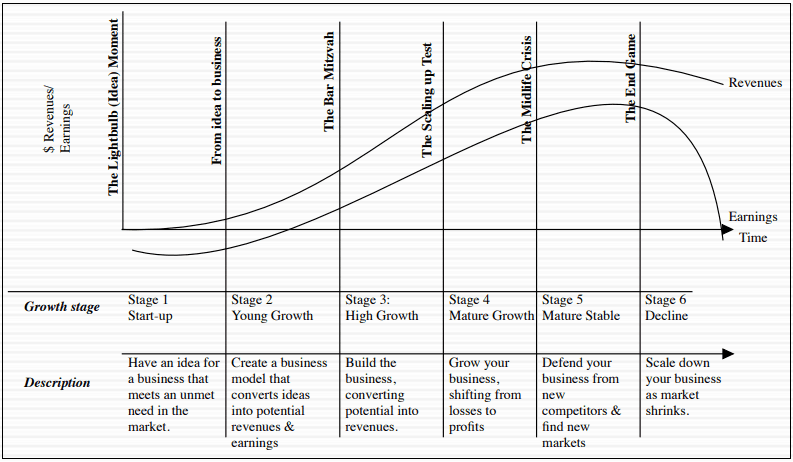Chart 1: Corporate Life Cycle.   Source: Damodaran

In this model, growth companies are those that have gone beyond the start-up stage but have yet to reach the mature stage. It is relatively easier to identify the mature stage. This is one where the growth rate is at best the GDP growth rate.

You generally rely on the revenue pattern to identify companies in this growth stage.

The challenge for valuing companies at the growth stage is projecting the duration and the growth rates. Damodaran suggests that you need a narrative to help you do this. He then uses a DCF valuation approach to value such companies.

In real life, some established companies continue to deliver high growth rates. In the Damodaran corporate life cycle framework, you would classify them as being in the high-growth stage.

Bruce Greenwald believes that this sustainable growth characteristic is only applicable to "franchises". These are companies with some economic moat that enables them to sustain high growth rates.

He suggests an Asset Value vs Earnings Power Value comparison (AV-EPV comparison) to identify them. High-growth companies are those where the EPV far exceeds the Asset Value. He then verifies them by looking at their economic moats or competitive advantages.

According to Greenwald, the high growth duration depends on the strength of the moat. One way to gauge this is to see how long it would take for the competitive advantage to compete away.

If a company has moats that can provide "protection" for more than 2 or 3 decades, then it makes sense to determine the value of growth.

However, Greenwald believes that there are too many uncertainties when using a DCF model. Instead, he suggests that you look at the return space rather than the value space when figuring out the margin of safety.

## DCF models

The intrinsic value of a company can be considered as the present value of the cash flow generated by a company over its life. It is obvious that to value a company, you have to first determine the cash flow for the various stages.

Then to determine the present value of the cash flow, you have to discount them using different discount rates for each stage of the life cycle. This is because the discount rate not only reflects the time value of money but also the risk associated with the cash flow. The risks would be different for different stages.

For valuing a start-up, you need the valuation metrics for the high-growth stage, the mature stage, and the decline stage. This is where the multi-stage valuation model comes in.

I use 2 valuation models for such situations. They are based on 2 valuation approaches - the discounted Free Cash Flow to the Firm (FCFF) model and the discounted Residual Income one.

With EXCEL, you can build a financial model covering the various stages and then use it to estimate the present value of all the cash flows. For both models, you have to add the non-operating assets and deduct Debt and minority interests to get the intrinsic value to equity holders.

### FCFF model

In the discounted FCFF approach, the cash flow here is the FCFF defined as:

FCFF = EBIT(1-t) × (1-Re).

Where

EBIT = earnings before interest and taxes.

t = tax rate.

Re = Reinvestment rate.

### Residual Income Model

In the Residual Income approach, the intrinsic value of the firm is the Total Capital Employed (TCE) plus the present value of the Residual Income.

Residual Income (RI) is defined as the after-tax EBIT minus a Capital charge. The relevant cash flow here is the Residual Income.

TCE = Shareholders Funds + Minority Interests + Debt - Cash & Other Non-operating Assets.

For consistency, the TCE should be the capital employed to generate the EBIT.

The Capital charge is represented by WACC × TCE.

RI = EBIT(1-t) - (WACC x TCE).

If your assumptions are consistent, both the FCFF and RI valuation models will give you the same answer.

In practice, the model I used for a company depends on the information available. I prefer the Residual Income model as it breaks down the firm value into 2 components. The first component is one due to the Book Value while the other is due to excess earnings.

## My CBIP Investment

I first invested in CBIP in 2017. I had considered this a compounder based on the following:
• From 2007 to 2016 revenue grew at 8.0 % CAGR and PAT at 10.2 % CAGR.
• ROE averaged 20.6 % from 2007 to 2016.
• Shareholders’ funds grew at 17. % CAGR from 2007 to 2016. This was mainly from retained earnings. While there were several rounds of bonus issues, there was no call for additional paid-up capital.

I would not go into the details on why I think CBIP is a good company here. Refer to my blog article if you want the details.

I use CBIP as the case study to illustrate the various computations. Table 2 summarizes the key parameters required for the valuations. They cover the key value drivers as per Damodaran.
• Revenue and the growth rates.
• Gross profit and SGA margins. I also assumed that these would change over time.
• Capital efficiency as measured by the TCE/Revenue and the growth rates.
• Some measures of how to fund the growth. I generally use the Reinvestment rates.
• The discount rates. I have derived the Cost of Equity and the WACC based on the Damodaran build-up method.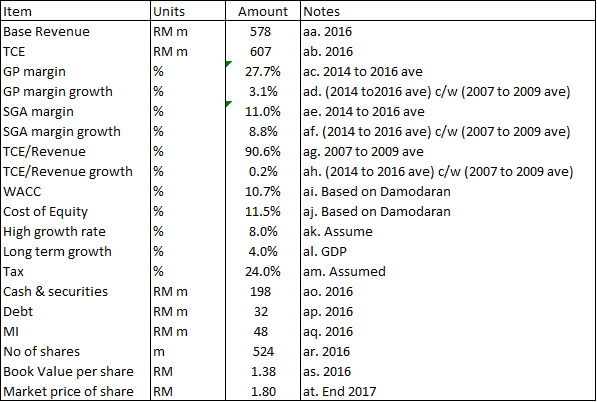Table 1: CBIP Parameters

## Worked examples – DCF

For this case study, I will assume that there is some growth narrative that matches the numbers shown in Table 1. I will not cover the narrative but focus on the computation aspects.

I will illustrate how I determined the intrinsic value of CBIP using both the FCFF model as well as the Residual income model.

### FCFF valuation

I used a 2-stage FCFF model to value CBIP where there is a high revenue growth stage followed by a lower long-term revenue growth rate.

Table 2 and the accompanying notes illustrate the computation.

I assumed that the high revenue growth stage to be 5 years. The model assumes that the low-growth stage continues in perpetuity. In other words, I do not factor any decline.

I have also assumed growth for others parameters – margins and capital efficiency. If you look at columns b, c, and f for the various years, you can see the changes.

The key parameters for each stage are the FCFF and the WACC. The FCFF for each stage in turn depends on the EBIT, tax rate, and Reinvestment rate.

As in my earlier article, I assumed that growth is determined by fundamentals.

g = Return × Reinvestment rate.

Return = EBIT(1-t)/TCE.  Note that I used the TCE of the previous period.

So if I can determine the TCE for each year I can then determine the Return for each year. Thereafter I can determine the Reinvestment rate for each year.

Conceptually I am determining the FCFF for each period and then discounting it to the present value ie year = 0.

The intrinsic value is the sum of all the individual discounted values. Note that the value of the mature stage is also discounted to the present value.

Based on this model, the intrinsic value of CBIP is RM 1.81 per share compared to the market price of RM 1.80 per share.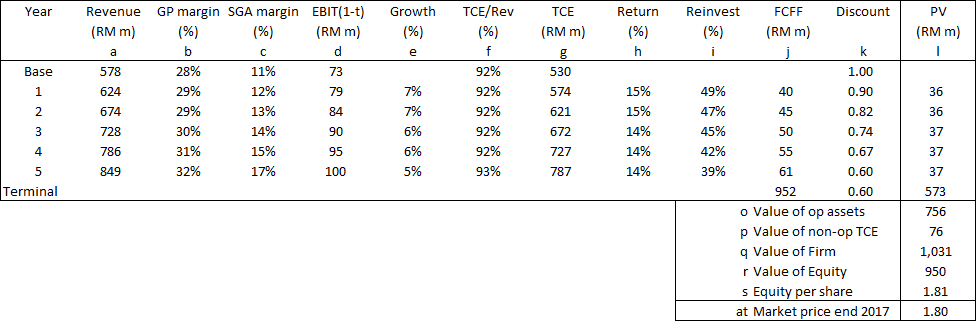Table 2: FCFF Valuation of CBIPNotes. Their alphabets refer to those in Tables 2 to 4. a) Revenue = Previous year revenue X (1 + high growth rate). b) GP margin = Previous year GP margin X (1 + GP margin growth rate). c) SGA margin = Previous year SGA margin X (1 + SGA margin growth rate). d) EBIT(1-t) = a(b-c) X (1 - tax rate). e) Growth = [ Current EBIT(1-t) / Previous year EBIT(1-t) ] - 1. f) TCE/Revenue = Previous year TCE/Revenue x (1 + TCE/Revenue growth rate). g) TCE = a X f. h) Return = EBIT(1-t) / TCE of the previous period. i) Reinvestment rate = Growth / Return. j) FCFF = EBIT(1-t) X (1 - Reinvestment rate). k) Discount factor = Previous year factor / (1 + WACC). l) Present value = FCFF X Discount factor. m) Capital charge = TCE of the previous period X WACC. n) Residual income = EBIT(1-t) - Capital charge. o) Value of op assets = Sum of PV including those from the Terminal Value . p) Value of non-op TCE. This is to account for the difference between the actual TCE and the one used in the model. q) Value of Firm = Value of op assets + Cash + Value of non-op TCE. r) Value of Equity = Value of firm - Debt - MI. s) Equity per share = Value of Equity / Number of shares.

### Residual Income valuation.

In this model, the key parameters for each year are the TCE and the WACC. This will enable us to determine the Residual Income for each year.

Table 3 illustrates the computation. I have assumed the same parameters for CBIP as those in the FCFF valuation example.

Based on the TCE/Revenue ratio, I then projected the TCE for each year. Given the WACC, I then determined the Capital charge for each year. Note that I used the TCE for the previous period to determine the current period Capital charge.

I then determined the Residual Income and the corresponding Terminal Residual Income. I determined the Terminal Residual Income using the perpetual growth formula.

The value of the operating assets to the Firm = current TCE + total present of the Residual Income.

Based on this model, the intrinsic value of CBIP is RM 1.94 per share compared to RM 1.81 per share from the FCFF model.

In theory, both approaches should give the same answer if the assumptions are consistent. The difference meant that I had some inconsistencies in my assumptions. In practice, I seldom get the same answers and so I generally take the average of both models as the final intrinsic value.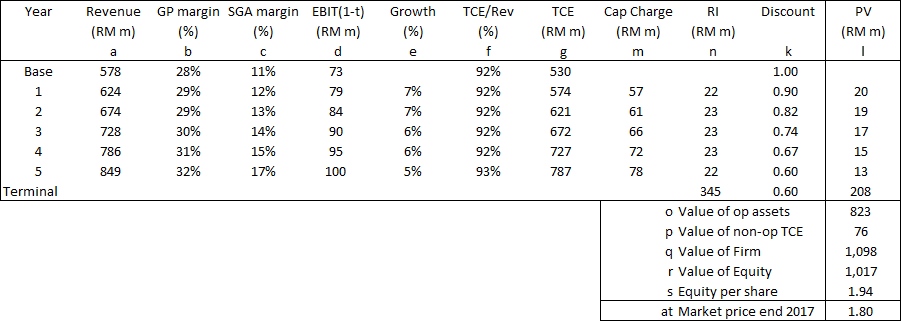Table 3: Residual Income Valuation of CBIPRefer to the Notes in Table 2 for the explanations of each of the alphabet notes.
If I assumed that all the growths – revenue, margins, capital efficiency – are zero, we have the Earnings Power Value (EPV). This is RM 1.68 per share.

Case Notes

In my CBIP examples, I have used what is known as a 2-stage model. It assumes that revenue will grow at one constant rate and then suddenly drop to a lower rate. Refer to the top image in Chart 2.

There is also another model known as the H model where the growth rate drops gradually over time. Refer to the bottom image in Chart 2.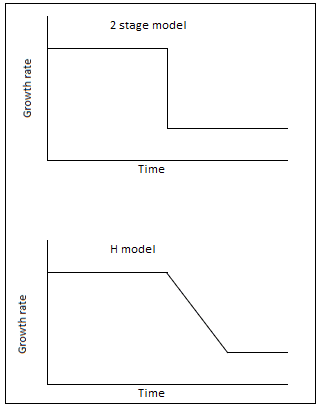Chart 2: Growth Models

There are standard formulae to determine the intrinsic values for these models. In the past, it was difficult to calculate the intrinsic values for other growth patterns. But with EXCEL, this is not an issue anymore.

In my DCF examples, I have different growth patterns for revenue, margins, and capital efficiency. I then computed the present values of the FCFF from first principles.

The challenge then is not the computation or the model. It is whether the model and assumptions reflect reality. You have to rely on your company analysis for this. As a sanity check, I compare my analysis with what others have done. I used sites such as Seeking Alpha* for this. Click the link for some free stock examples. If you subscribe to their services, you can tap into their business analysis and valuation.

## Greenwald Return approach

There are 3 steps in Greenwald approach:
• Using the AV-EPV comparison to identify the high-growth companies.
• Verifying then with competitive analysis.
• Determining the Returns.

The EPV of CBIP is RM 1.68 per share. The Asset Value (refer to Book Value in Table 1) = RM 1.38 per share.

According to Greenwald, EPV > AV can only be sustainable if there is some moat. In my CBIP article, I mentioned that CBIP has some patents for its technology. This is certainly a form of a moat.

Moats and competitive advantages are topics that are better covered in MBA-type resources. Refer to "Baby Steps into the Investment Universe" if you want to dig deeper.

My point is that CBIP looks like a compounder ie a company with the potential to compound its return. The challenge then is whether there is a margin of safety based on Greenwald’s Return approach

As for the Returns, Greenwald classifies them into 3:
• Cash returns. These are from Dividends and buybacks.
• Returns from reinvestments. For this to have value, the return has to be greater than the cost of capital.
• Organic growth This comes from the general economic growth and Greenwood considers them as "free".

Greenwald formula contains 3 components. The first component is for the cash returns. The second component refers to the returns from reinvestments. The last is for organic growth.

Expected return = b(E/P) + (1-b)(E/P)(ROE/r) + g

Where:

b = portion of earnings distributed.

E = Earnings per share.

P = Price per share.

ROE = Return on Equity

r = Cost of Equity.

g = growth.

Based on the historical data, you determined the amount set aside for Dividends and share buybacks.

You then estimate the Return from this component by dividing it by the EPS. This is your first Return component. Let us assume that this is Y %.

You next estimate what is available for Reinvestment by deducting the portion for Dividends and share buybacks. You then determine the reinvested Return. This is not merely dividing by the EPS. Let us assume that the return with this simple division is Z %.

Rather he estimates whether the reinvested Return would be higher or lower than Z %. He does this by comparing the marginal return on capital with the marginal cost of capital.

For example, if the return is 15% compared to the 10% cost of capital, it means that for every dollar invested, the company gets 1.5 dollars of return. He then assumed the reinvested Return to be 1.5Z %.

From what I could see, Greenwald considers that there is no need for any investment to generate organic growth.

However, instead of using the long-term GDP growth rate as the organic return, he makes certain adjustments.
• He adds 1% if the company serves the luxury market.
• He deducts 3% if the company serves the mass market.
• He deducts 0.5 % if it sells goods rather than services.

In his example, the long-term US GDP growth rate of 4 % translates to 0.05 % organic Return after the above deductions.

We now have the 3 components to estimate the total Return = cash Return + reinvested Return + organic growth.

In our example, this = (Y + 1.5Z + 0.05) %.

Greenwald then compares this with the cost of capital to look for the margin of safety.

The amount of margin of safety should be linked to the strength of the moat. If you have a moat that takes a decade to be eroded, you want a higher margin than one with 5 decades of protection.

### Limitations of the Return approach

According to Greenwald, the main problem with the Return approach is that there is no dollar estimate of the intrinsic value. As such it is more difficult to determine when it is overvalued.

According to Greenwald, investors such as Warren Buffet and Seth Klarman set some guidelines. Warren Buffet supposedly holds the stocks forever. Seth Klarman has some PE cut-offs.

I would have thought that a reasonable sell guideline would be when the total Return is half of the cost of capital. Unfortunately, I do not have sufficient such types of investments to see whether this guideline is workable.

### Organic Return

There are 2 issues here:
• What is the organic return?
• What is the Reinvestment required for the organic return?

I have discussed how Greenwald treated the organic return as one with zero reinvestment. But then he adjusts the long-term GDP growth rate to derive the organic growth rate.

I have seen a Greenwald article where he used the long-term growth rates in productivity and profit margin as the free organic return.

The logic of the free organic return can be seen from the dividend discount model.

P = D/(r - g).

Return = D/P + g.

But I saw an article where some reinvestment was deducted to get the return from organic growth.

If you look at the Greenwald return formula, you can see that the organic growth rate can have a significant impact on the total return. As such I would take a conservative estimate and not use the full GDP growth rate.

Damodaran has suggested that the long-term growth rate for the terminal value be set at the risk-free rate. I think this is a good rate.

The best way is to think of the growth that can be achieved without any investment. Examples are:
• If you are a retailer, this could be same-store sales.
• Improvement in operating margins by being more efficient.
• Changes to higher margin product mix to get better margins.

## Worked example - Greenwald Return

In this section, I illustrate how I used the Greenwald approach to determine the return for CBIP using the following formula.

Return = b(E/P) + (1-b)(E/P)(ROE/r) + g

Table 4 illustrates how I derived the values for each component.

Based on this approach, I estimated the total return = 15.1 %. If I compare this with the Cost of Equity of 11.5 %, there is a 30.8 % margin of safety.

On this basis, I concluded that there is a margin of safety. This was even though there is no margin of safety based on the DCF approach.

In my work example, I have assumed that organic growth is the GDP growth rate.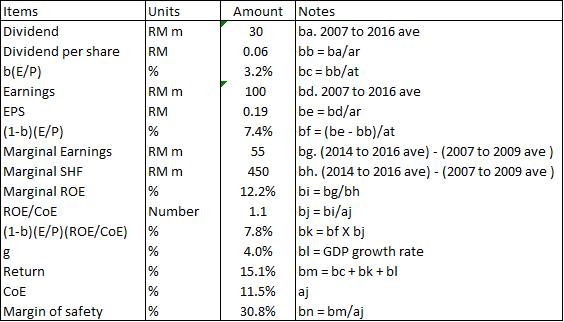Table 4: Estimating the Returns for CBIP based on Greenwald MethodNotes: Refer to the alphabet notes for the steps in the calculation. For an explanation of some of the alphabet notes, refer to the notes in Table 2.

## High-growth cyclical companies

Cyclical companies are those whose performance varies with some macroeconomic or commodity factors.

Because of the cyclical nature, extrapolating from the current position may lead to a wrong picture. To overcome this, we should normalize the performance. To account for the changes in size, we start with the current revenue. We then determine the earnings and FCFF with the normalized margins and reinvestment rates.

Cyclical companies can also be high-growth ones. The chart below illustrates the difference between a cyclical company with growth and one without. The green line shows a cyclical pattern with growth while the blue line shows the one without growth.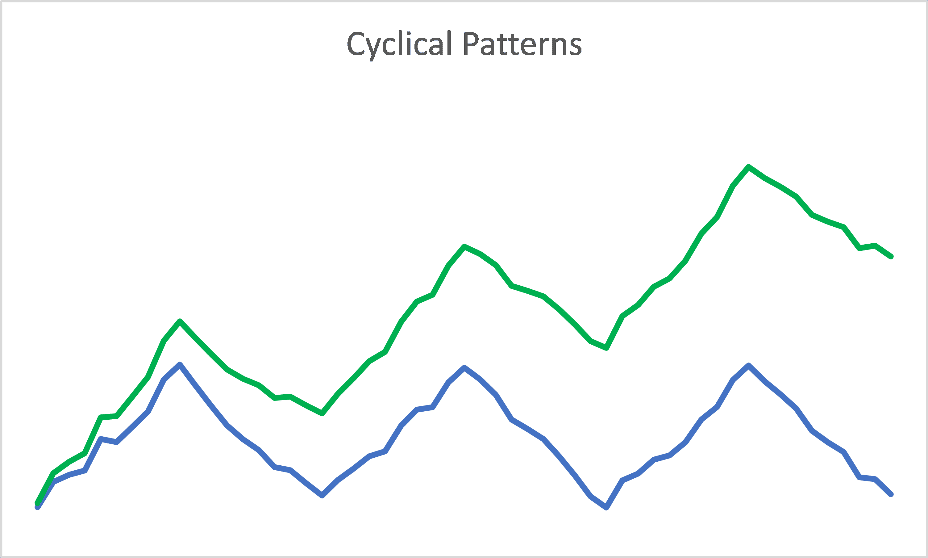Chart 3: No growth cyclical pattern c/w cyclical pattern with growth

The challenge is when there are growths in the margins and reinvestment rates. A good example is illustrated in Chart 4. Using just the normalized margins does not capture the improvements over time. To account for these improvements, I scaled the normalized values.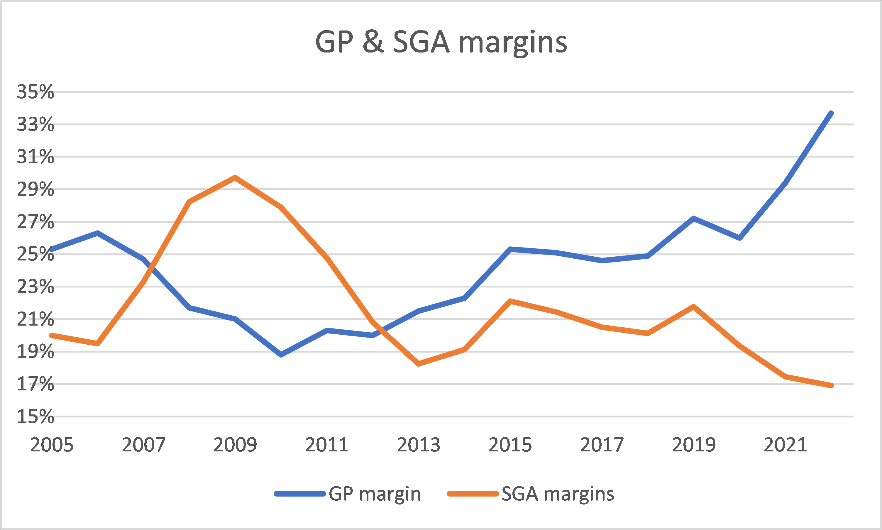Chart 4: Improvements in Margins

In my BlueLinx analysis, as shown in Table 5, I derived a scaling factor by comparing the current peak value with the previous peak value.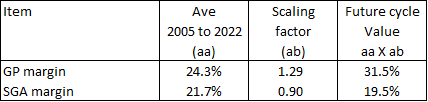Table 5: Scaling Factor

It is straightforward to use the normalized and scaled values for the Damodaran approaches. You using the current revenue and scaled margins and capital efficiencies as base values.

But when it comes to Greenwald, high growth is not specifically part of the return formula.

Return = b(E/P) + (1-b)(E/P)(ROE/r) + g

Note that the g in the formula represents the organic growth and not the high growth.

You have to account for the high growth in the improvements in the margins and reinvestment rates. You do this by determining the current earnings (E) based on the current revenue and the scaled margins. Conceptually this is no different from determining the scaled earnings for the DCF models.

You also have to scale the dividends so that b(E/P) represents the scaled value. Next, you should also use the scaled earnings to determine the ROE. This will ensure internal consistency in your formula.

## Conclusion

Damodaran and Greenwald have proposed different valuation approaches for high-growth companies.

Damodaran approach with the narrative and FCFF model applies to companies coming out of the start-up stage. With these companies, there is hardly any operating history. At the same time, the lack of historical data may make AV-EPV comparison difficult.

Greenwald approach is more suitable for established companies with growth rates far over the GDP growth rates. His AV-EPV comparison is a good starting point to identify such high-growth companies. You then verify them by identifying the competitive advantages.

Note that in Greenwald’s approach, the high growth rate is not the main metric. Greenwald then looks at the return space to determine the margin of safety.

I hope I have made a case for both to be in your valuation toolbox. I generally do not invest in companies just exiting the start-up stage. I am more likely to invest in established high-growth companies. So, you should not be surprised that I consider the Greenwald approach more appropriate.